# NCERT Solutions For Class 10 Maths Chapter 3 Exercise 3.3 – Pair of Linear Equations in two Variables

Download NCERT Solutions For Class 10 Maths Chapter 3 Exercise 3.3 – Pair of Linear Equations in two Variables. This Exercise contains 3 questions, for which detailed answers have been provided in this note. In case you are looking at studying the remaining Exercise for Class 10 for Maths NCERT solutions for other Chapters, you can click the link at the end of this Note.

### NCERT Solutions For Class 10 Maths Chapter 3 Exercise 3.3 – Pair of Linear Equations in two Variables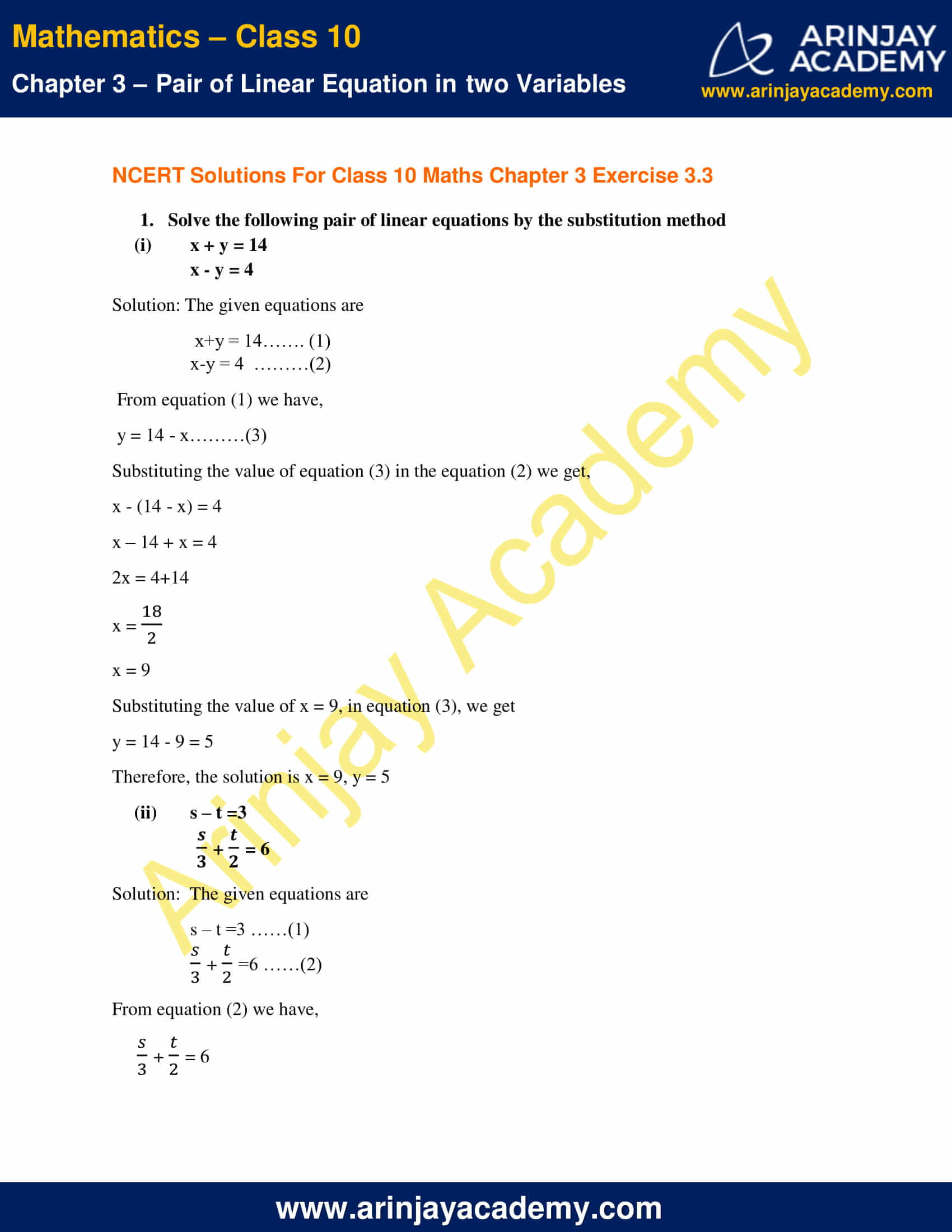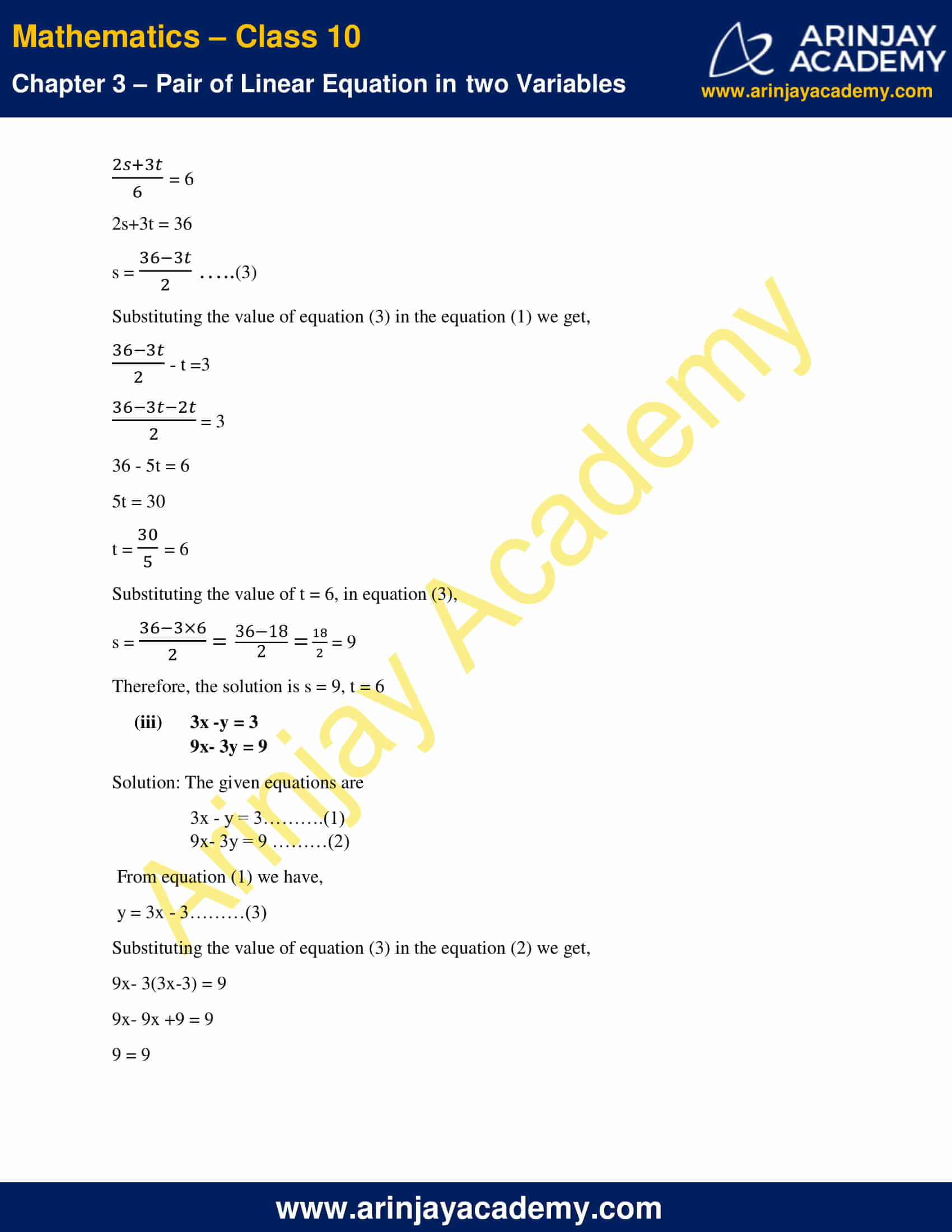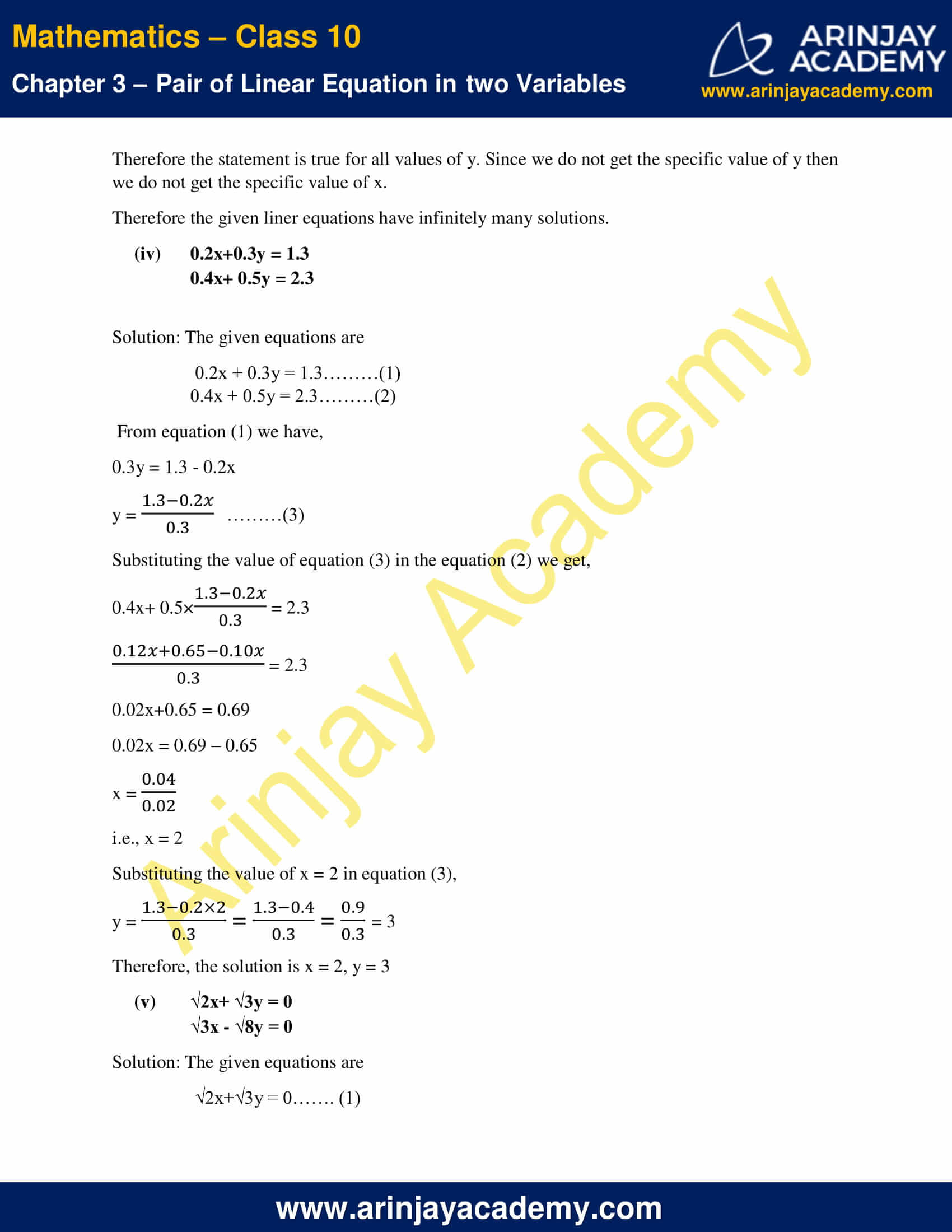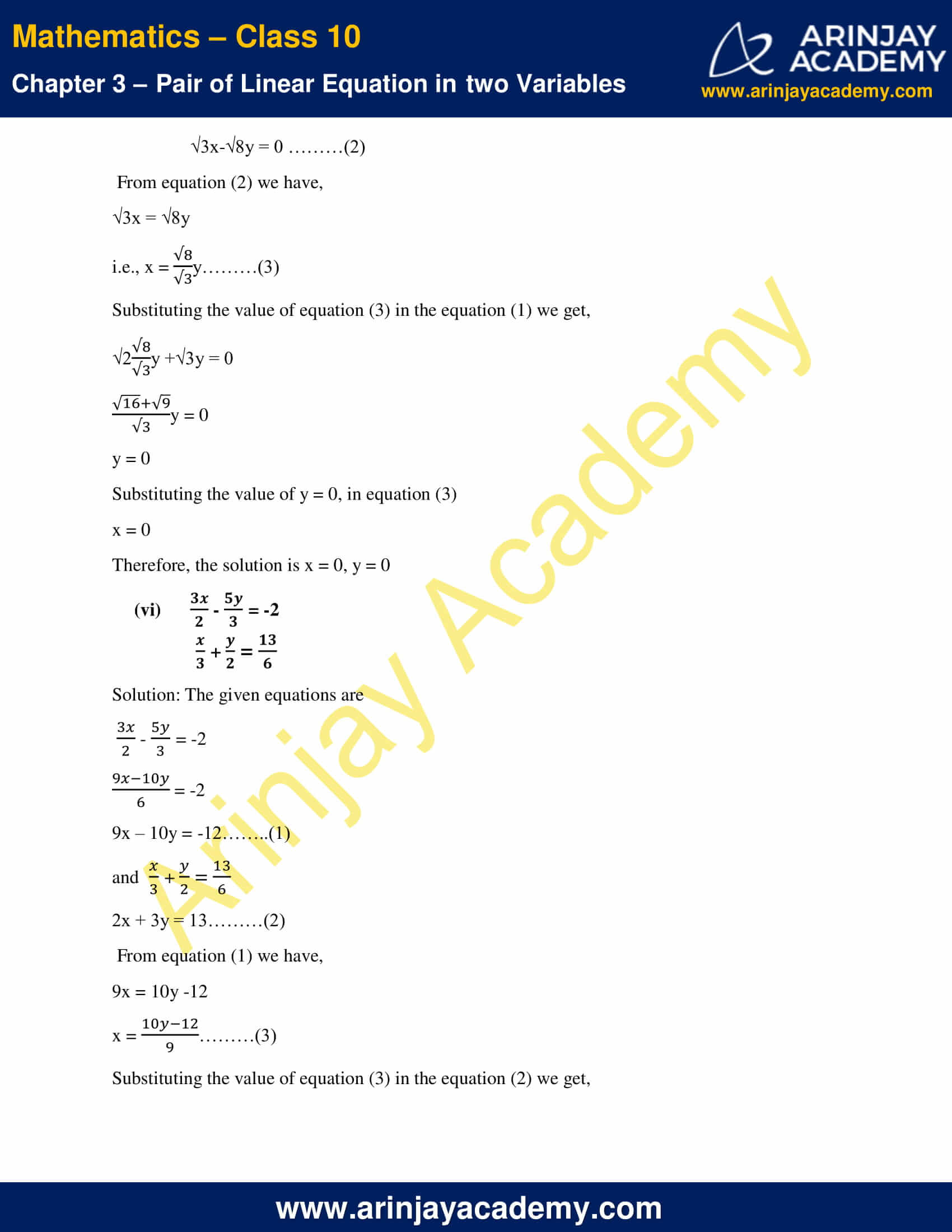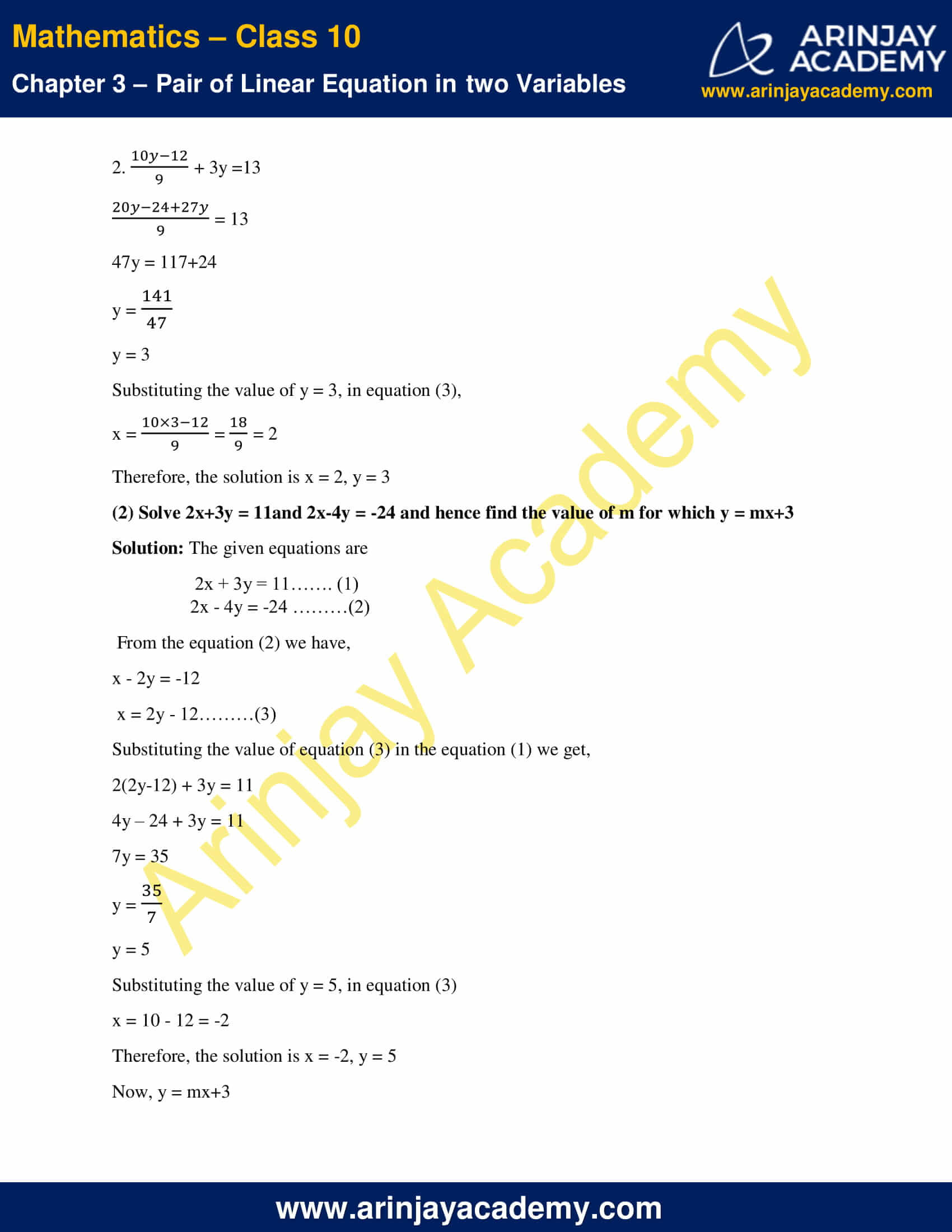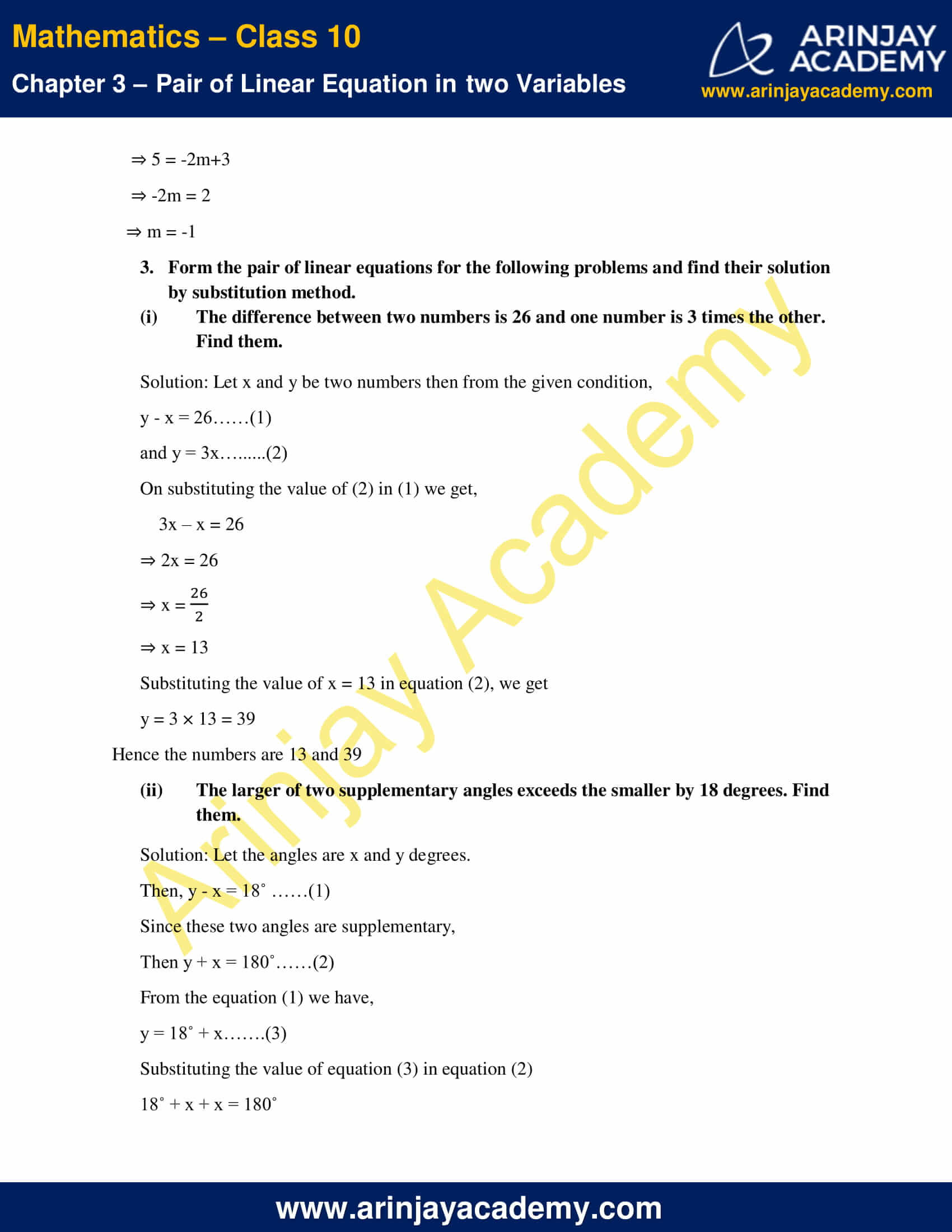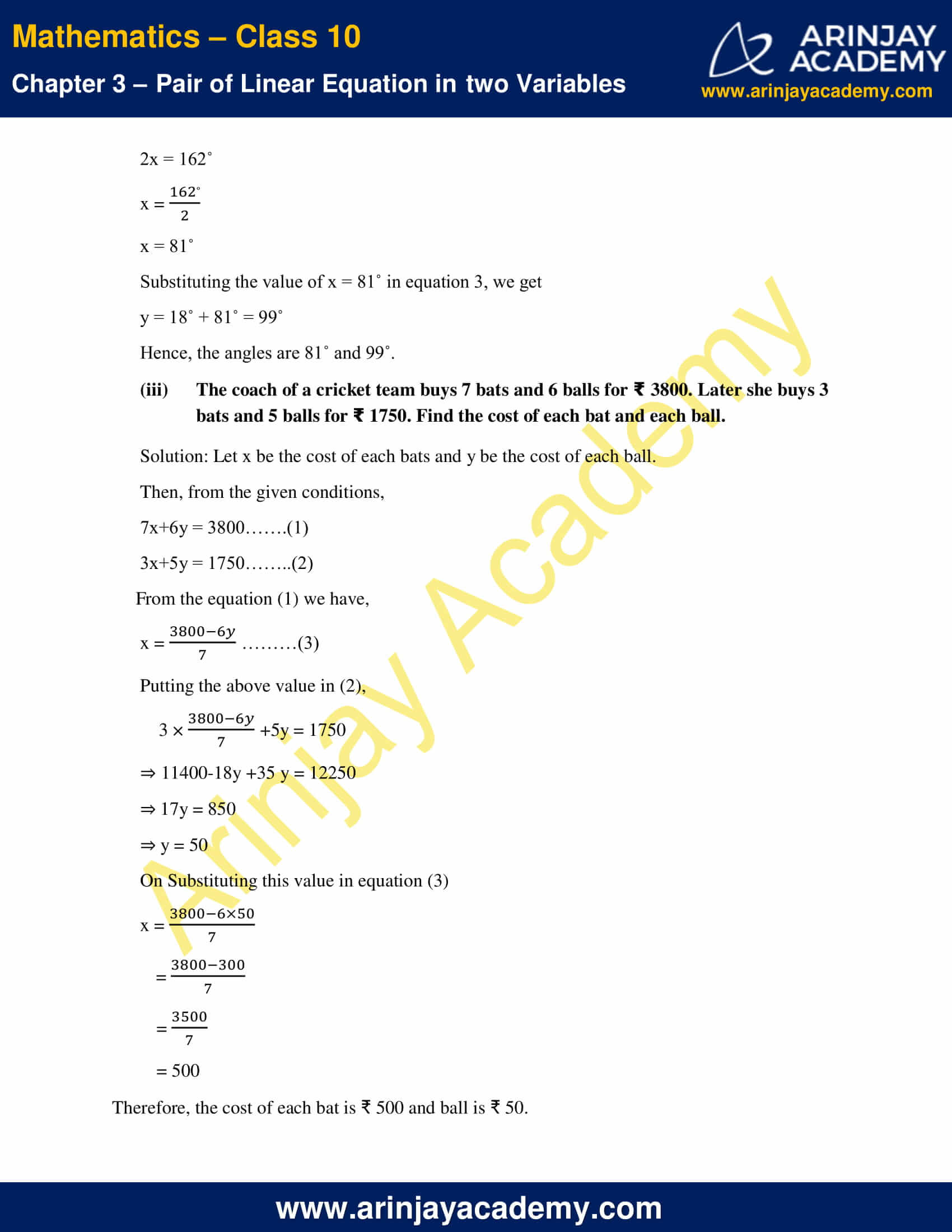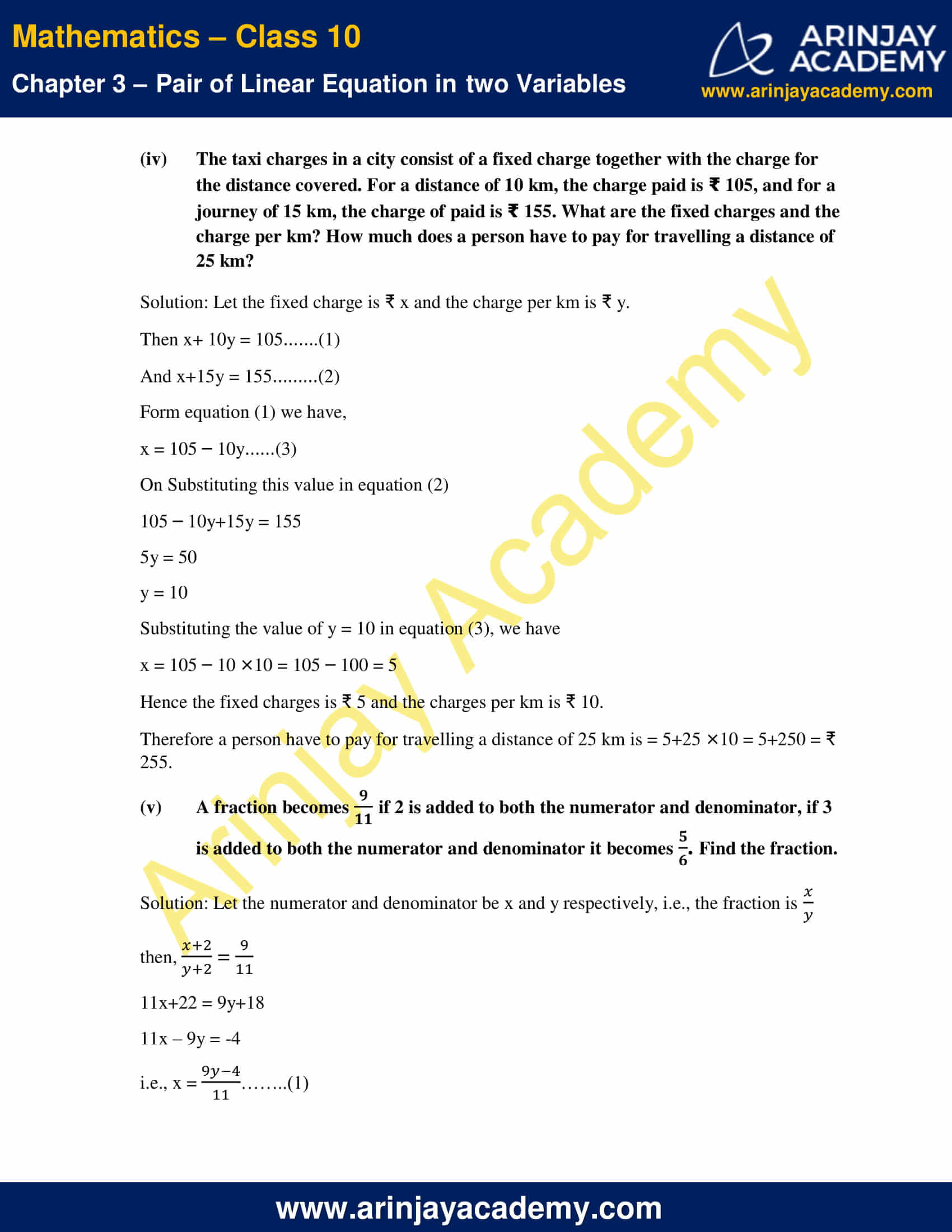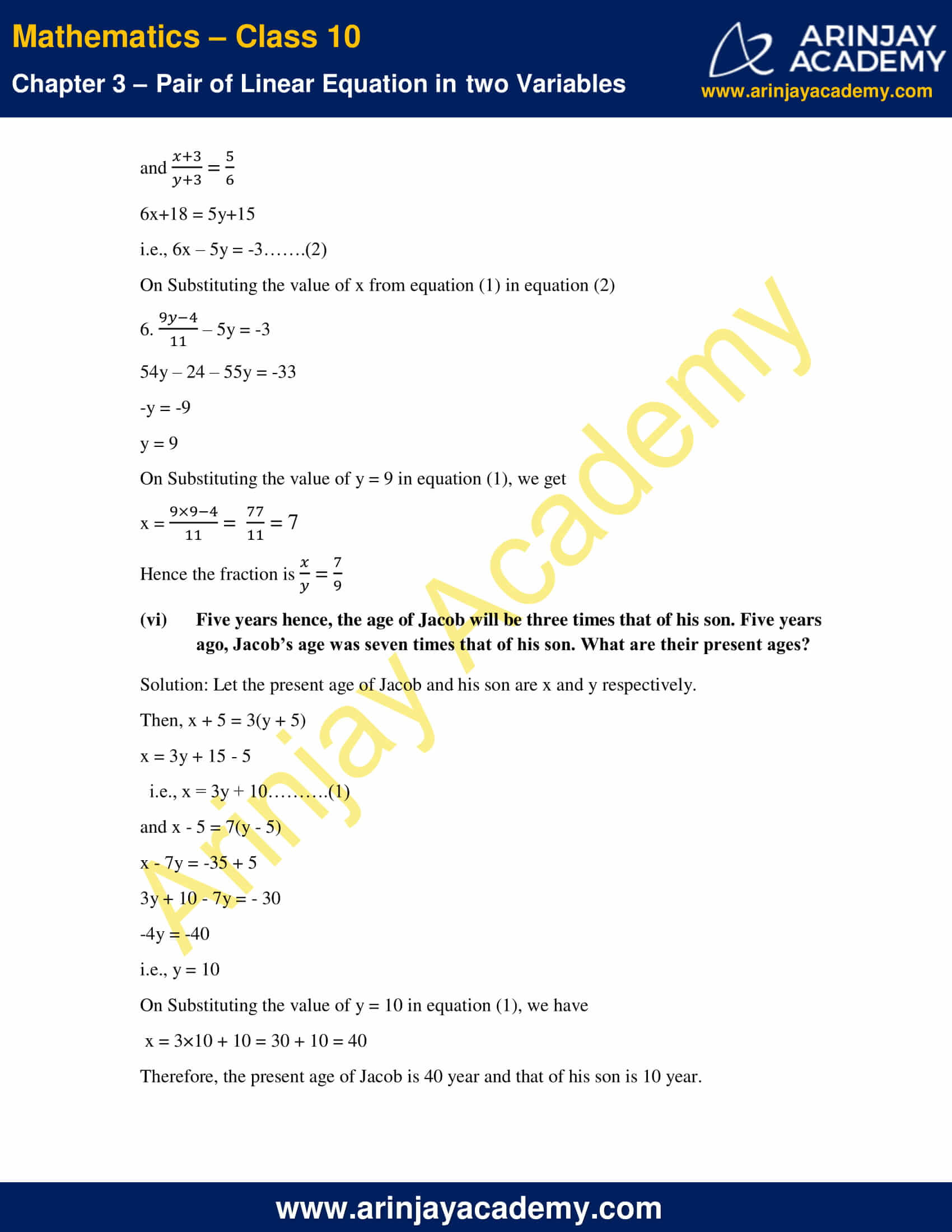NCERT Solutions For Class 10 Maths Chapter 3 Exercise 3.3 – Pair of Linear Equations in two Variables

1. Solve the following pair of linear equations by the substitution method

(i) x + y = 14

x – y = 4

Solution:

The given equations are

x+y = 14……. (1)

x-y = 4  ………(2)

From equation (1) we have,

y = 14 – x………(3)

Substituting the value of equation (3) in the equation (2) we get,

x – (14 – x) = 4

x – 14 + x = 4

2x = 4+14

x =$\cfrac { 18 }{ 2 }$

x = 9

Substituting the value of x = 9, in equation (3), we get

y = 14 – 9 = 5

Therefore, the solution is x = 9, y = 5

(ii) s – t =3$\cfrac { s }{ 3 }$ +$\cfrac { t }{ 2 }$  = 6

Solution:

The given equations are

s – t =3 ……(1)$\cfrac { s }{ 3 }$ +$\cfrac { t }{ 2 }$ = 6 ……(2)

From equation (2) we have,$\cfrac { s }{ 3 }$ +$\cfrac { t }{ 2 }$ = 6$\cfrac { 2s+3t }{ 6 }$ = 6

2s+3t = 36

s =$\cfrac { 36-3t }{ 2 }$…..(3)

Substituting the value of equation (3) in the equation (1) we get,$\cfrac { 36-3t }{ 2 }$ – t =3$\cfrac { 36-3t-2t }{ 2 }$ = 3

36 – 5t = 6

5t = 30

t =$\cfrac { 30 }{ 5 }$ = 6

Substituting the value of t = 6, in equation (3),

s =$\cfrac { 36-3\times 6 }{ 2 }$ =$\cfrac { 36-18 }{ 2 }$=$\cfrac { 18 }{ 2 }$= 9

Therefore, the solution is s = 9, t = 6

(iii) 3x -y = 3

9x- 3y = 9

Solution:

The given equations are

3x – y = 3……….(1)

9x- 3y = 9 ………(2)

From equation (1) we have,

y = 3x – 3………(3)

Substituting the value of equation (3) in the equation (2) we get,

9x- 3(3x-3) = 9

9x- 9x +9 = 9

9 = 9

Therefore the statement is true for all values of y. Since we do not get the specific value of y then we do not get the specific value of x.

Therefore the given liner equations have infinitely many solutions.

(iv) 2x+0.3y = 1.3

0.4x+ 0.5y = 2.3

Solution:

The given equations are

0.2x + 0.3y = 1.3………(1)

0.4x + 0.5y = 2.3………(2)

From equation (1) we have,

0.3y = 1.3 – 0.2x

y =$\cfrac { 1.3-0.2x }{ 0.3 }$………(3)

Substituting the value of equation (3) in the equation (2) we get,

0.4x+ 0.5×$\cfrac { 1.3-0.2x }{ 0.3 }$  = 2.3$\cfrac { 0.12x+0.65-0.10x }{ 0.3 }$ = 2.3

0.02x+0.65 = 0.69

0.02x = 0.69 – 0.65

x =$\cfrac { 0.04 }{ 0.02 }$

i.e., x = 2

Substituting the value of x = 2 in equation (3),

y =$\cfrac { 1.3-0.2\times 2 }{ 0.3 }$ =$\cfrac { 1.3-0.4 }{ 0.3 }$ =$\cfrac { 0.9 }{ 0.3 }$ = 3

Therefore, the solution is x = 2, y = 3

(v) √2x+ √3y = 0

√3x – √8y = 0

Solution:

The given equations are

√2x+√3y = 0……. (1)

√3x-√8y = 0 ………(2)

From equation (2) we have,

√3x = √8y

i.e., x =$\cfrac { \sqrt { 8 } }{ \sqrt { 3 } }$y………(3)

Substituting the value of equation (3) in the equation (1) we get,

√2.$\cfrac { \sqrt { 8 } }{ \sqrt { 3 } }$ y +√3y = 0$\cfrac { \sqrt { 16 } +\sqrt { 9 } }{ \sqrt { 3 } }$y = 0

y = 0

Substituting the value of y = 0, in equation (3)

x = 0

Therefore, the solution is x = 0, y = 0

(vi)$\cfrac { 3x }{ 2 }$$\cfrac { 5y }{ 3 }$  = -2$\cfrac { x }{ 3 }$ +$\cfrac { y }{ 2 }$  =$\cfrac { 13 }{ 6 }$

Solution:

The given equations are$\cfrac { 3x }{ 2 }$$\cfrac { 5y }{ 3 }$  = -2$\cfrac { 9x-10y }{ 6 }$ = -2

9x – 10y = -12……..(1)

and$\cfrac { x }{ 3 }$ +$\cfrac { y }{ 2 }$ =$\cfrac { 13 }{ 6 }$

2x + 3y = 13………(2)

From equation (1) we have,

9x = 10y -12

x =$\cfrac { 10y-12 }{ 9 }$………(3)

Substituting the value of equation (3) in the equation (2) we get,

2.$\cfrac { 10y-12 }{ 9 }$+ 3y =13$\cfrac { 20y-24+27y }{ 9 }$ = 13

47y = 117+24

y =$\cfrac { 141 }{ 47 }$

y = 3

Substituting the value of y = 3, in equation (3),

x =$\cfrac { 10\times 3-12 }{ 9 }$ =$\cfrac { 18 }{ 9 }$ = 2

Therefore, the solution is x = 2, y = 3

2. Solve 2x+3y = 11and 2x-4y = -24 and hence find the value of m for which y = mx+3

Solution:

The given equations are

2x + 3y = 11……. (1)

2x – 4y = -24 ………(2)

From the equation (2) we have,

x – 2y = -12

x = 2y – 12………(3)

Substituting the value of equation (3) in the equation (1) we get,

2(2y-12) + 3y = 11

4y – 24 + 3y = 11

7y = 35

y =$\cfrac { 35 }{ 7 }$

y = 5

Substituting the value of y = 5, in equation (3)

x = 10 – 12 = -2

Therefore, the solution is x = -2, y = 5

Now, y = mx+3

⇒ 5 = -2m+3

⇒ -2m = 2

⇒ m = -1

3. Form the pair of linear equations for the following problems and find their solution by substitution method.

(i) The difference between two numbers is 26 and one number is 3 times the other. Find them.

Solution: Let x and y be two numbers then from the given condition,

y – x = 26……(1)

and y = 3x………(2)

On substituting the value of (2) in (1) we get,

3x – x = 26

⇒ 2x = 26

⇒ x =$\cfrac { 26 }{ 2 }$

⇒ x = 13

Substituting the value of x = 13 in equation (2), we get

y = 3 × 13 = 39

Hence the numbers are 13 and 39

(ii) The larger of two supplementary angles exceeds the smaller by 18 degrees. Find them.

Solution: Let the angles are x and y degrees.

Then, y – x = 18˚ ……(1)

Since these two angles are supplementary,

Then y + x = 180˚……(2)

From the equation (1) we have,

y = 18˚ + x…….(3)

Substituting the value of equation (3) in equation (2)

18˚ + x + x = 180˚

2x = 162˚

x =$\cfrac { 162 }{ 2 }$

x = 81˚

Substituting the value of x = 81˚ in equation 3, we get

y = 18˚ + 81˚ = 99˚

Hence, the angles are 81˚ and 99˚.

(iii) The coach of a cricket team buys 7 bats and 6 balls for Later she buys 3 bats and 5 balls for 1750. Find the cost of each bat and each ball.

Solution: Let x be the cost of each bats and y be the cost of each ball.

Then, from the given conditions,

7x+6y = 3800…….(1)

3x+5y = 1750……..(2)

From the equation (1) we have,

x =$\cfrac { 3800-6y }{ 7 }$………(3)

Putting the above value in (2),

3 ×$\cfrac { 3800-6y }{ 7 }$  +5y = 1750

⇒ 11400-18y +35 y = 12250

⇒ 17y = 850

⇒ y = 50

On Substituting this value in equation (3)

x =$\cfrac { 3800-6\times 50 }{ 7 }$

=$\cfrac { 3800-300 }{ 7 }$

=$\cfrac { 3500 }{ 7 }$

= 500

Therefore, the cost of each bat is ₹ 500 and ball is ₹ 50.

(iv) The taxi charges in a city consist of a fixed charge together with the charge for the distance covered. For a distance of 10 km, the charge paid is 105, and for a journey of 15 km, the charge of paid is What are the fixed charges and the charge per km? How much does a person have to pay for travelling a distance of 25 km?

Solution: Let the fixed charge is ₹ x and the charge per km is ₹ y.

Then x+ 10y = 105…….(1)

And x+15y = 155………(2)

Form equation (1) we have,

x = 105 – 10y……(3)

On Substituting this value in equation (2)

105 – 10y+15y = 155

5y = 50

y = 10

Substituting the value of y = 10 in equation (3), we have

x = 105 – 10 ×10 = 105 – 100 = 5

Hence the fixed charges is ₹ 5 and the charges per km is ₹ 10.

Therefore a person have to pay for travelling a distance of 25 km is = 5+25 ×10 = 5+250 = ₹ 255.

(v) A fraction becomes if 2 is added to both the numerator and denominator, if 3 is added to both the numerator and denominator it becomes . Find the fraction.

Solution: Let the numerator and denominator be x and y respectively, i.e., the fraction is

then,$\cfrac { x+2 }{ y+2 }$ =$\cfrac { 9 }{ 11 }$

11x+22 = 9y+18

11x – 9y = -4

i.e., x =$\cfrac { 9y-4 }{ 11 }$……..(1)

and$\cfrac { x+3 }{ y+3 }$ =$\cfrac { 5 }{ 6 }$

6x+18 = 5y+15

i.e., 6x – 5y = -3…….(2)

On Substituting the value of x from equation (1) in equation (2)

6.$\cfrac { 9y-4 }{ 11 }$ – 5y = -3

54y – 24 – 55y = -33

-y = -9

y = 9

On Substituting the value of y = 9 in equation (1), we get

x =$\cfrac { 9\times 9-4 }{ 11 }$ =$\cfrac { 77 }{ 11 }$  = 7

Hence the fraction is$\cfrac { x }{ y }$ =$\cfrac { 7 }{ 9 }$

(vi) Five years hence, the age of Jacob will be three times that of his son. Five years ago, Jacob’s age was seven times that of his son. What are their present ages?

Solution: Let the present age of Jacob and his son are x and y respectively.

Then, x + 5 = 3(y + 5)

x = 3y + 15 – 5

i.e., x = 3y + 10……….(1)

and x – 5 = 7(y – 5)

x – 7y = -35 + 5

3y + 10 – 7y = – 30

-4y = -40

i.e., y = 10

On Substituting the value of y = 10 in equation (1), we have

x = 3×10 + 10 = 30 + 10 = 40

Therefore, the present age of Jacob is 40 year and that of his son is 10 year.

NCERT Solutions for Class 10 Maths Chapter 3 Exercise 3.3 – Pair of Linear Equations in two Variables, has been designed by the NCERT to test the knowledge of the student on the topic – Algebraic Methods of Solving a Pair of Linear Equations – Substitution Method

Download NCERT Solutions For Class 10 Maths Chapter 3 Exercise 3.3 – Pair of Linear Equations in two Variables